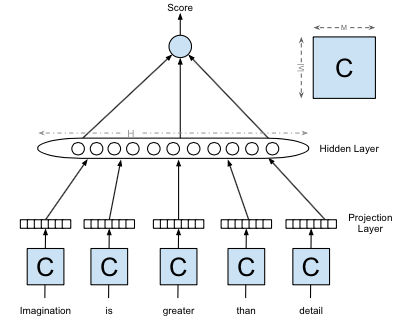# Polyglot: Distributed Word Representations for Multilingual NLP

Rami Al-Rfou, Bryan Perozzi, Steven Skiena (all at Stony Brook University)

Published in the proceedings of CoNLL 2013 (PDF).

The authors train word embeddings for 117 different languages using Wikipedia. The embeddings are trained using an architecture similar to that of SENNA of Collobert et al. This architecture computes a score representing the likelihood that the words given as input occurred together in order. A short window is scanned over a stream of text, and the score of the phrase in the window is compared to the score of a corrupted version of the same phrase where the middle word is substituted randomly. The model is penalised (using hinge loss, i.e. one-way error) according to whether the uncorrupted or corrupted phrase was more highly scored.

The score of a phrase is computed as per the following:1. Each of the words is transformed from a one-hot to a distributed representation via the application of a shared matrix $C$, and these representations are concatenated;
2. The hyperbolic tan of an affine transformation of this concatenation is calculated component-wise, yielding a “hidden” vector.
3. The components of this vector are combined via an affine transformation to yield the score.

So this neural network has three layers and the parameters are the shared matrix $C$ together with the two affine transformations.

The word embedding is given by the rows of the shared matrix $C$.

The models are trained using Theano for extensive periods of time (the authors mention “weeks”). The window size is taken to be radial length 2, the word embedding rank is 64 and the hidden layer size is 32.

To demonstrate the utility of the word representations, the authors the representations as initialisation for a model performing parts of speech tagging.

The paper was published at about the same time as word2vec (it does not refer to word2vec at all). The approach, the notation and the terminology, however, demonstrate that certain things that I had thought particular to word2vec were in fact already accepted practice, including:

• the use of discriminative tasks for training word embeddings
• sampling contexts by scanning a short window over text
• the use of the middle word in a context for the discriminative task
• dividing through by the “fan out” for initialisation (page 187, TBC)
• the symbols <S> and </S> for delimiting sentences